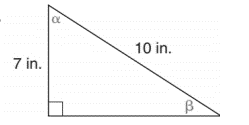Chapter 11.2, Problem 24E### Elementary Geometry for College St...

6th Edition
Daniel C. Alexander + 1 other
ISBN: 9781285195698

#### Solutions

Chapter
Section### Elementary Geometry for College St...

6th Edition
Daniel C. Alexander + 1 other
ISBN: 9781285195698
Textbook Problem
1 views

# In Exercise 23 to 28, use either the sine ratio or the cosine ratio as needed to find the measure of each indicated angle to the nearest degree.To determine

To find:

The measure of each indicated angle of the following triangle,Explanation

Consider the following figure,

General formula for cosine ratio and sin ratio is given below,

From the given figure the value 10 in. is the hypotenuse value since it is opposite to right angle.

Then 7 in. is the length of the leg adjacent to α and 7 in. is the length of leg opposite to β.

Therefore,

sinβ=710β=sin1(710)

Using a graphing calculator in degree mode, follow the following key sequence,

sin

### Still sussing out bartleby?

Check out a sample textbook solution.

See a sample solution

#### The Solution to Your Study Problems

Bartleby provides explanations to thousands of textbook problems written by our experts, many with advanced degrees!

Get Started

#### Find more solutions based on key concepts